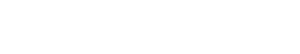# Regression

In the context of text analytics, regression is a technique used to predict a numeric value, such as a price or quantity. This prediction is based on historical data, which is used to build a model that can be applied to new data.

Regression can be used for different types of problems, such as predicting the amount of money a customer will spend, or the number of items a customer will purchase. In each case, the goal is to find the relationship between a set of input variables (such as product features) and the output variable (such as price or quantity).

There are many different types of regression models, and the choice of model depends on the nature of the data and the problem being solved. Some common types of regression models include linear regression, logistic regression, and multivariate adaptive regression splines (MARS).

## Regression in Statistics

Regression is a statistical technique used to quantify the relationships between variables. In statistics, regression is used to predict future values of a variable based on past values of that same variable.

Two basic Types of Regression:

• Linear Regression: In linear regression, the relationship between the dependent and independent variables is represented by a straight line.
• Multiple Linear Regression: In multiple linear regression, the relationship between the dependent and independent variables is represented by a straight line that is composed of multiple line segments.

## Regression vs. Correlation

It is important to note that regression is different from correlation. Correlation is a measure of the relationship between two variables, while regression is a technique for predicting a numeric value.

In general, correlation is used to determine whether two variables are related, and if so, how strong that relationship is. Correlation can be positive or negative, and it can be weak or strong.

For example, you might use correlation to determine whether there is a relationship between the amount of time spent studying and the grade received on a test. In this case, you would expect to see a positive correlation, because as the amount of time spent studying increases, the grade received on the test is also likely to increase.

You can use regression to predict the grade received on a test based on the amount of time spent studying, but you can also use regression to predict the amount of time spent studying based on the grade received on the test. In other words, regression can be used to find the relationship between two variables, just like correlation.

The difference is that regression can be used to predict a numeric value, while correlation can only be used to determine whether two variables are related.

### One thought on “Regression”

1.mplrs.com says:

Thanks again.Unlock the power of actionable insights with AI-based natural language processing.